# CH 6 STACKS QUEUES AND DEQUES ACKNOWLEDGEMENT THESE

• Slides: 41CH 6. STACKS, QUEUES, AND DEQUES ACKNOWLEDGEMENT: THESE SLIDES ARE ADAPTED FROM SLIDES PROVIDED WITH DATA STRUCTURES AND ALGORITHMS IN JAVA, GOODRICH, TAMASSIA AND GOLDWASSER (WILEY 2016)ABSTRACT DATA TYPES (ADTS) • • An abstract data type (ADT) is an abstraction of a data structure An ADT specifies: • • • Data stored Operations on the data Error conditions associated with operations • Example: ADT modeling a simple stock trading system • • • The data stored are buy/sell orders The operations supported are • • order buy(stock, shares, price) • void cancel(order) order sell(stock, shares, price) Error conditions: • • Buy/sell a nonexistent stock Cancel a nonexistent order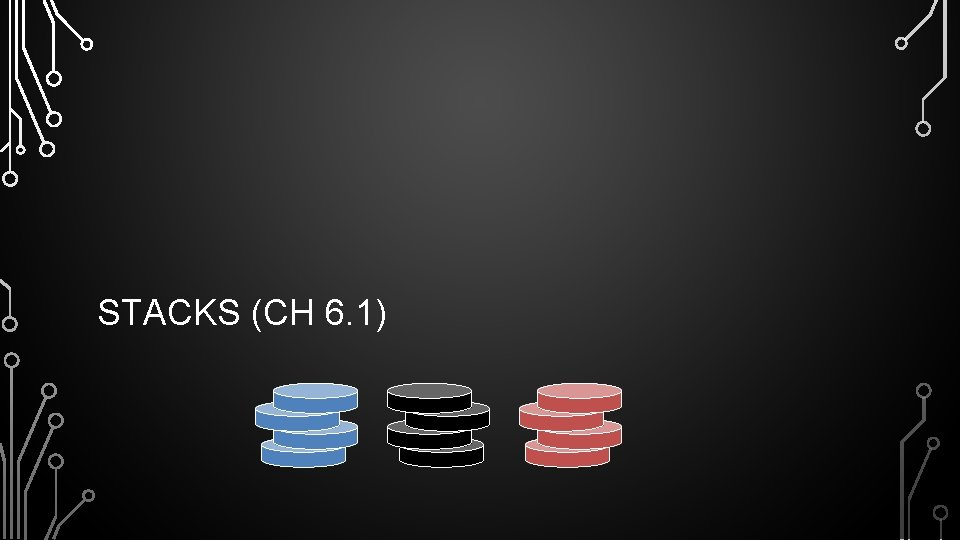STACKS (CH 6. 1)STACKS • A data structure similar to a neat stack of something, basically only access to top element is allowed – also reffered to as LIFO (last-in, first-out) storage • Direct applications • • • Page-visited history in a Web browser Undo sequence in a text editor Chain of method calls in the Java Virtual Machine • Indirect applications • • Auxiliary data structure for algorithms Component of other data structuresEXAMPLE STACK METHOD STACK IN THE JVM • The Java Virtual Machine (JVM) • • keeps track of the chain of active methods with a stack When a methods is called, the JVM pushes on the stack a frame containing local variables and return value When a method ends, its frame is popped from the stack and control is passed to the method on top of the stack main() { int i = 5; foo(i); } bar m=6 foo(int j) { int k = j+1; bar(k); } foo bar(int m) { … } main j=5 k=6 i=5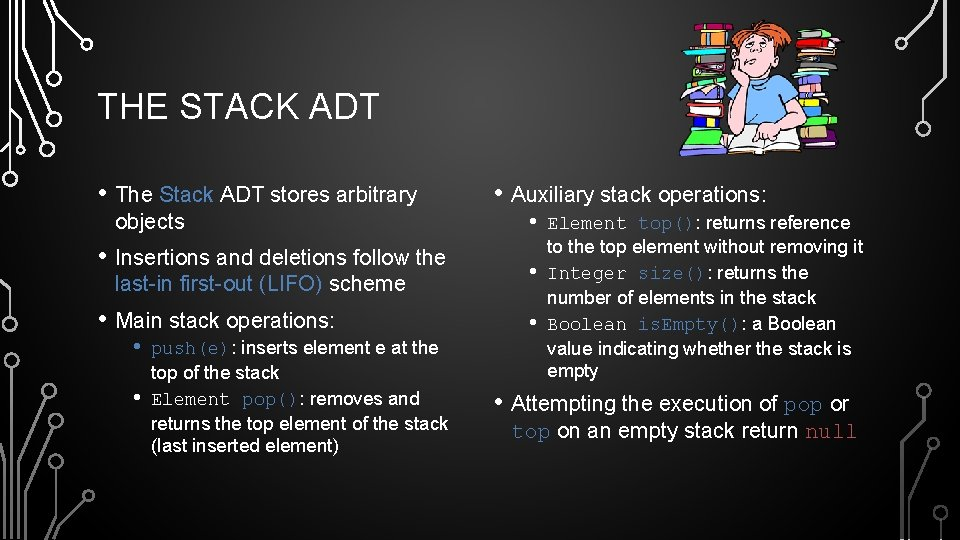THE STACK ADT • The Stack ADT stores arbitrary objects • Insertions and deletions follow the last-in first-out (LIFO) scheme • Main stack operations: • • push(e): inserts element e at the top of the stack Element pop(): removes and returns the top element of the stack (last inserted element) • Auxiliary stack operations: • • • Element top(): returns reference to the top element without removing it Integer size(): returns the number of elements in the stack Boolean is. Empty(): a Boolean value indicating whether the stack is empty • Attempting the execution of pop or top on an empty stack return nullEXAMPLE STACK INTERFACE IN JAVA public interface Stack<E> { int size(); /** Return number of elements */ boolean is. Empty(); /** True is size is 0 */ E top(); /** Return visible stack element */ void push(E e); /** Add to the stack */ E pop(); /** Remove from the stack */ }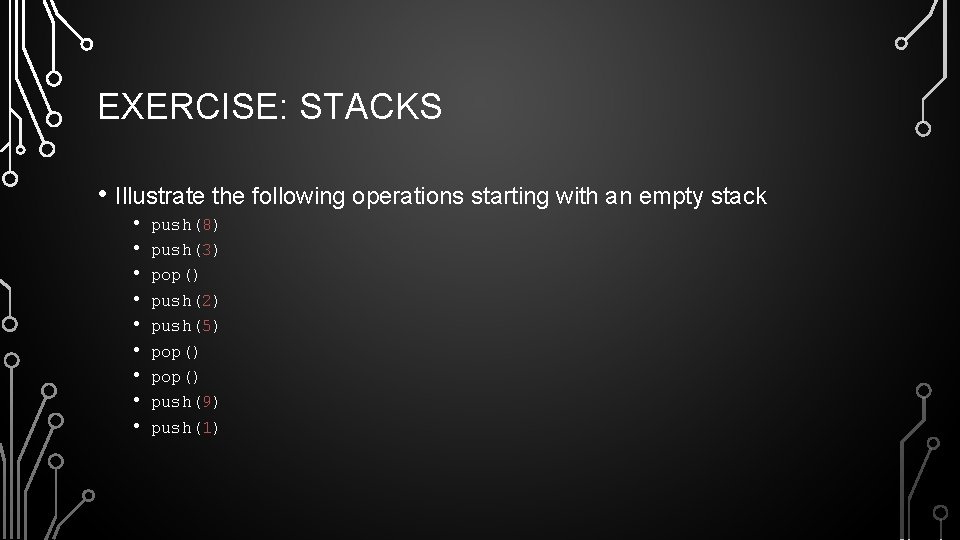EXERCISE: STACKS • Illustrate the following operations starting with an empty stack • • • push(8) push(3) pop() push(2) push(5) pop() push(9) push(1)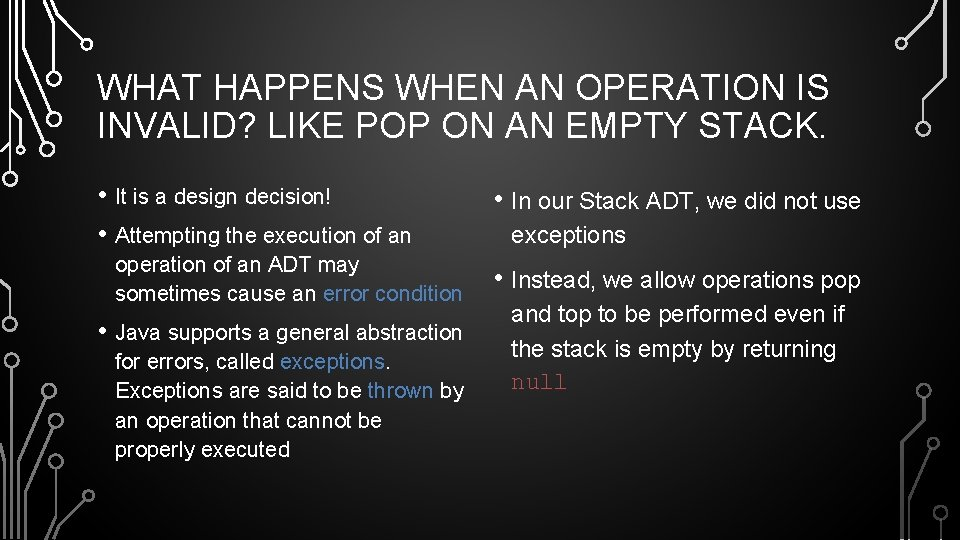WHAT HAPPENS WHEN AN OPERATION IS INVALID? LIKE POP ON AN EMPTY STACK. • It is a design decision! • Attempting the execution of an operation of an ADT may sometimes cause an error condition • Java supports a general abstraction for errors, called exceptions. Exceptions are said to be thrown by an operation that cannot be properly executed • In our Stack ADT, we did not use exceptions • Instead, we allow operations pop and top to be performed even if the stack is empty by returning nullARRAY-BASED STACK • A simple way of implementing the • • Stack ADT uses an array We add elements from left to right A variable keeps track of the index of the top element • … S 0 1 2 t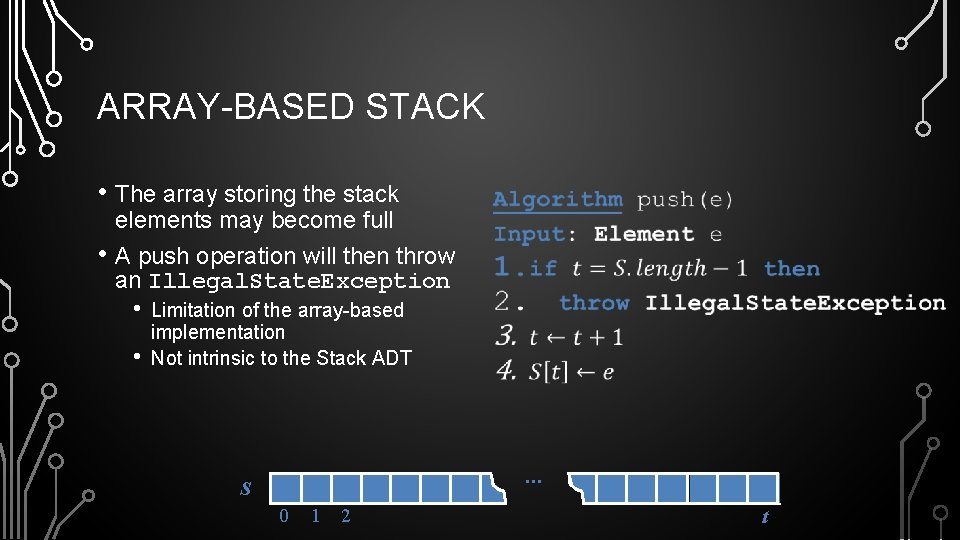ARRAY-BASED STACK • The array storing the stack • elements may become full A push operation will then throw an Illegal. State. Exception • • • Limitation of the array-based implementation Not intrinsic to the Stack ADT … S 0 1 2 tJAVA IMPLEMENTATION public class Array. Stack<E> implements Stack<E> { private E[] stack; private int t = -1; public void push(E e) throws Illegal. State. Exception { if(size() == stack. length) throw Illegal. State. Exception( "Stack is full"); stack[++t] = e; } public Array. Stack() {this(10); } public Array. Stack(int capacity) { stack = (E[]) new Object[capacity]; } public E pop() { if(is. Empty()) return null; E e = stack[t]; stack[t--] = null; return e; } public int size() {return t + 1; } public boolean is. Empty() {return t == -1; } public E top() { if(is. Empty()) return null; return stack[t]; } }PERFORMANCE AND LIMITATIONS ARRAY-BASED IMPLEMENTATION •GROWABLE ARRAY-BASED STACK • •COMPARISON OF THE STRATEGIES • AMORTIZATION MEANS PAYING OFF AN AMOUNT OVER TIME, NOT AVERAGE. THIS ANALYSIS RECOGNIZES EACH OPERATION IS INHERENTLY UNEQUAL. IT IS A STANDARD COMPUTER SCIENCE METHOD TO DEAL WITH THIS.INCREMENTAL STRATEGY ANALYSIS •DOUBLING STRATEGY ANALYSIS • 2 4 1 1 8EXERCISE STACK WITH A SINGLY-LINKED LIST •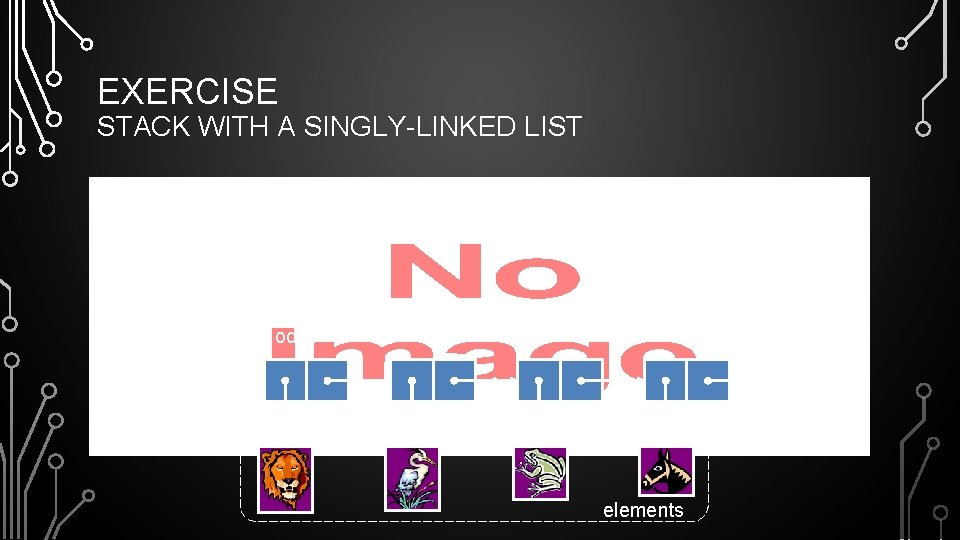EXERCISE STACK WITH A SINGLY-LINKED LIST • nodes top elementsSTACK SUMMARY Array Fixed-Size pop() push(o) top() size(), empty() Array Expandable (doubling strategy) List Singly-LinkedQUEUES (CH 6. 2)APPLICATIONS OF QUEUES • Direct applications • • • Waiting lines Access to shared resources (e. g. , printer) Multiprogramming • Indirect applications • • Auxiliary data structure for algorithms Component of other data structuresTHE QUEUE ADT • • Auxiliary queue operations: • • • Element first(): returns the element at the front without removing it Integer size(): returns the number of elements stored Boolean is. Empty(): indicates whether no elements are stored • Boundary cases • Attempting the execution of dequeue or first on an empty queue returns null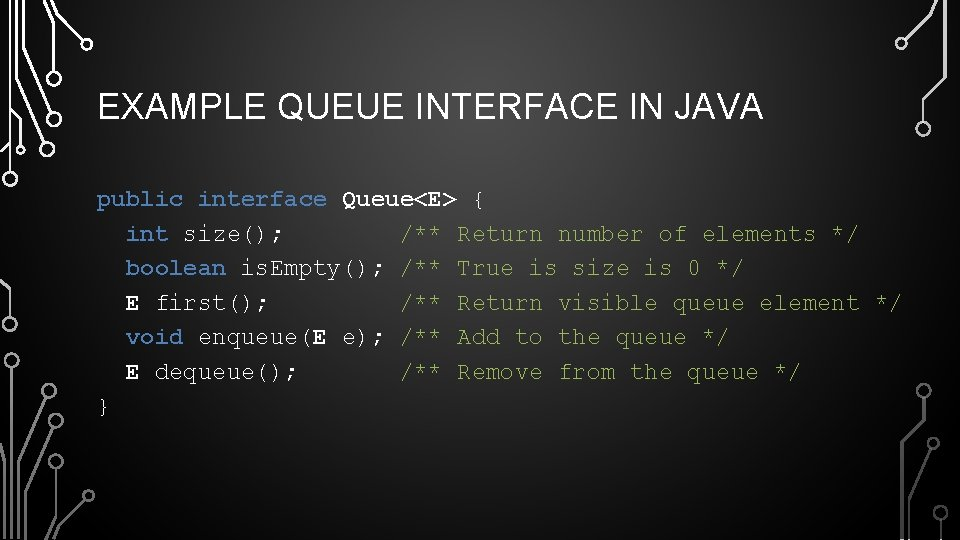EXAMPLE QUEUE INTERFACE IN JAVA public interface Queue<E> { int size(); /** Return number of elements */ boolean is. Empty(); /** True is size is 0 */ E first(); /** Return visible queue element */ void enqueue(E e); /** Add to the queue */ E dequeue(); /** Remove from the queue */ }EXERCISE: QUEUES • Illustrate the following operations starting with an empty queue • • • enqueue(8) enqueue(3) dequeue() enqueue(2) enqueue(5) dequeue() enqueue(9) enqueue(1)ARRAY-BASED QUEUE • normal configuration Q 0 1 2 f wrapped-around configuration Q 0 1 2 f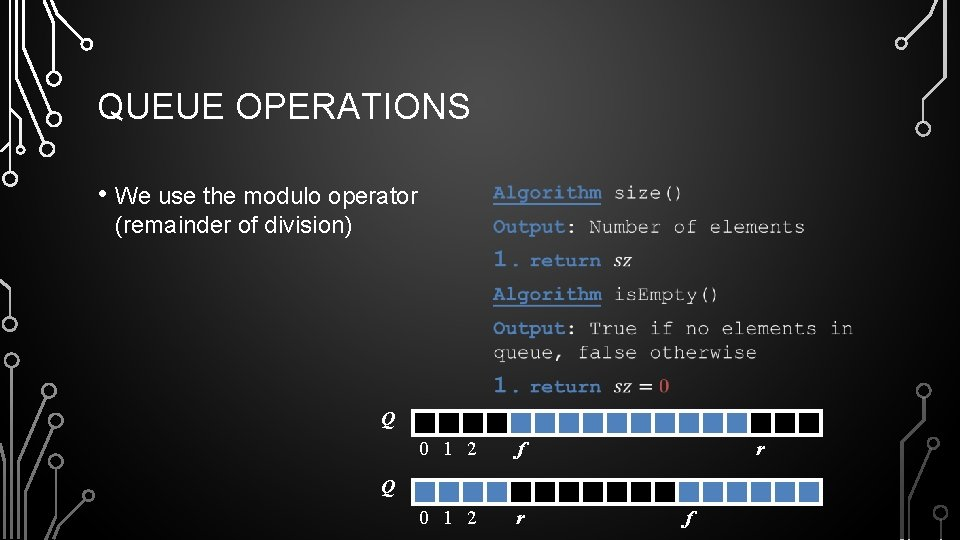QUEUE OPERATIONS • We use the modulo operator • (remainder of division) Q 0 1 2 f 0 1 2 r r Q f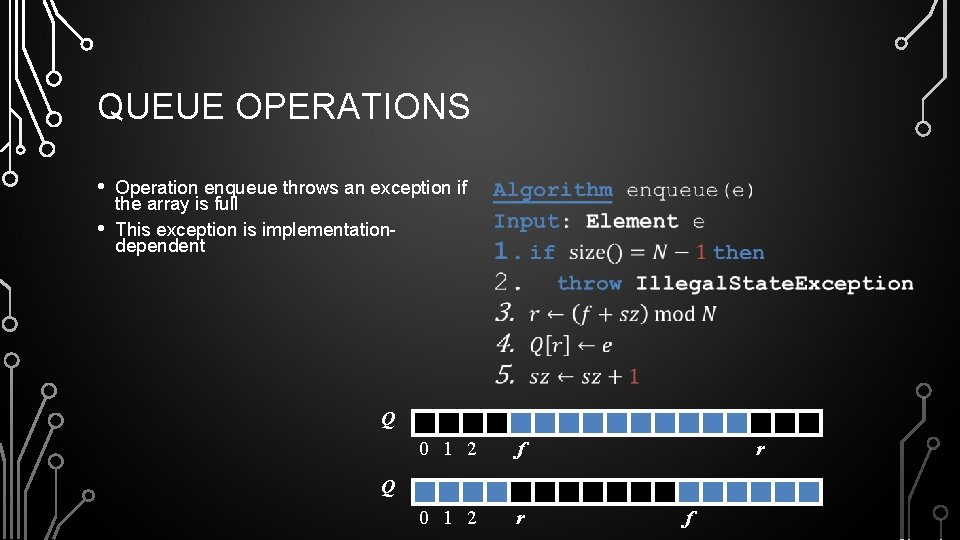QUEUE OPERATIONS • • Operation enqueue throws an exception if the array is full This exception is implementationdependent • Q 0 1 2 f 0 1 2 r r Q f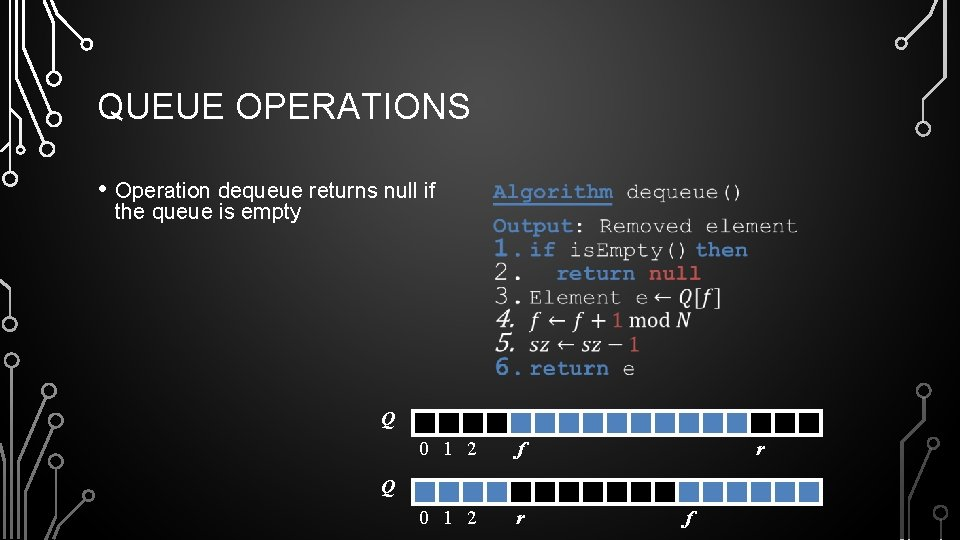QUEUE OPERATIONS • Operation dequeue returns null if the queue is empty • Q 0 1 2 f 0 1 2 r r Q f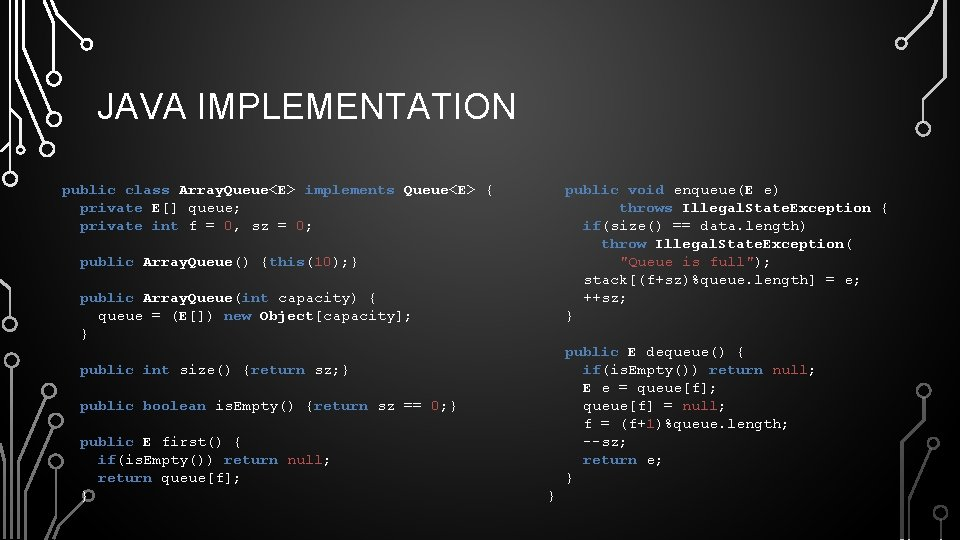JAVA IMPLEMENTATION public class Array. Queue<E> implements Queue<E> { private E[] queue; private int f = 0, sz = 0; public void enqueue(E e) throws Illegal. State. Exception { if(size() == data. length) throw Illegal. State. Exception( "Queue is full"); stack[(f+sz)%queue. length] = e; ++sz; } public Array. Queue() {this(10); } public Array. Queue(int capacity) { queue = (E[]) new Object[capacity]; } public E dequeue() { if(is. Empty()) return null; E e = queue[f]; queue[f] = null; f = (f+1)%queue. length; --sz; return e; } public int size() {return sz; } public boolean is. Empty() {return sz == 0; } public E first() { if(is. Empty()) return null; return queue[f]; } }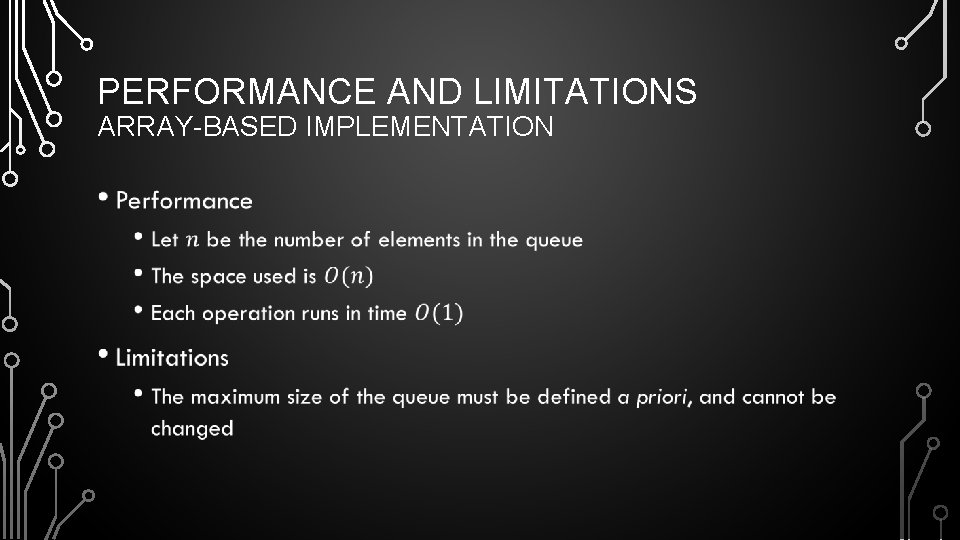PERFORMANCE AND LIMITATIONS ARRAY-BASED IMPLEMENTATION •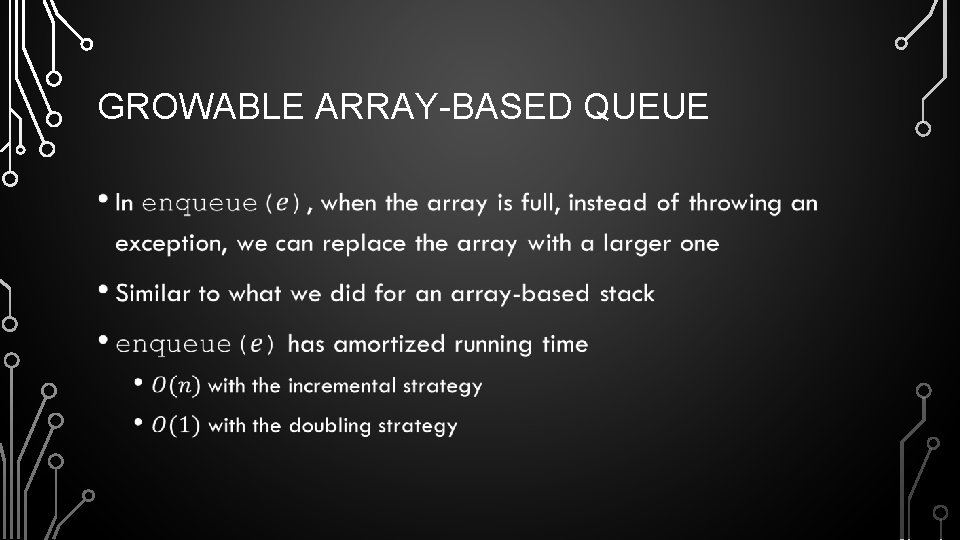GROWABLE ARRAY-BASED QUEUE •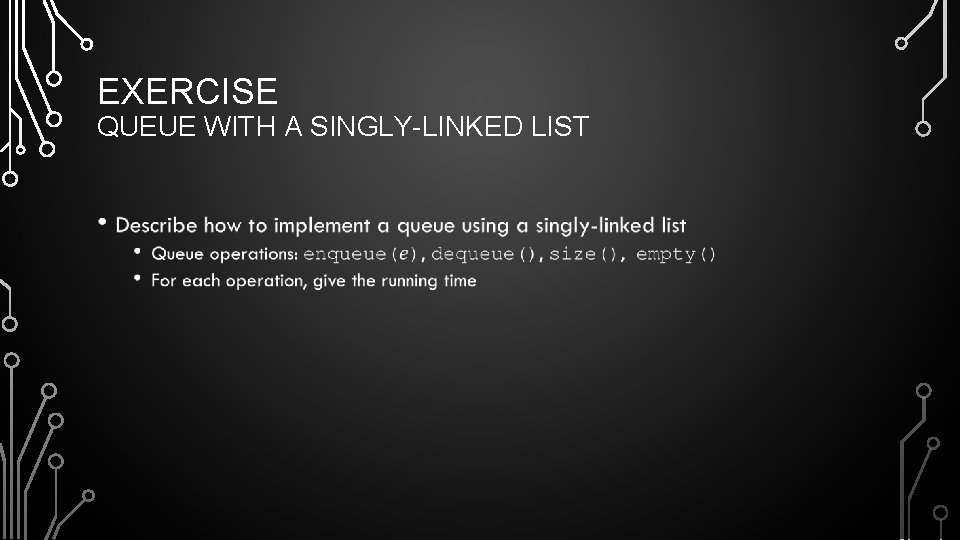EXERCISE QUEUE WITH A SINGLY-LINKED LIST •EXERCISE QUEUE WITH A SINGLY-LINKED LIST • r nodes first f elements rear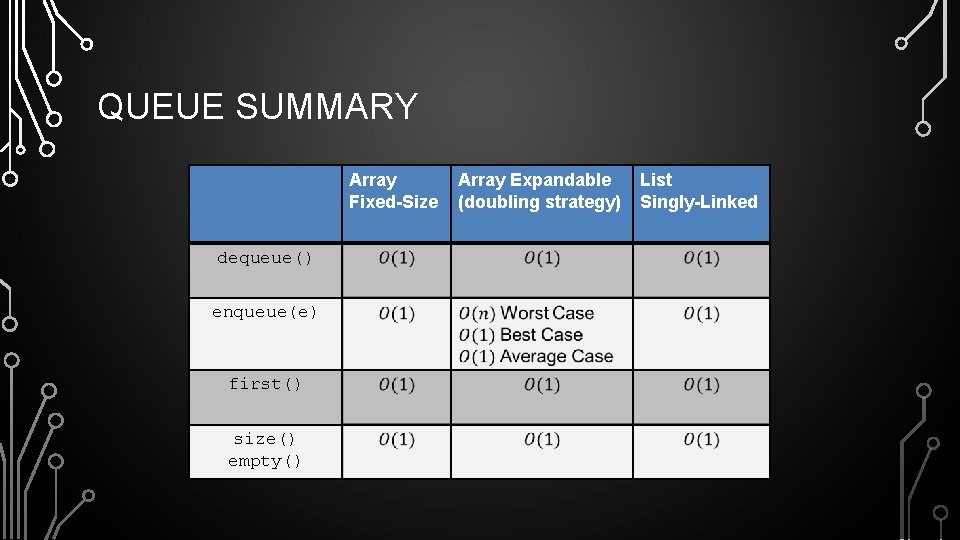QUEUE SUMMARY Array Fixed-Size dequeue() enqueue(e) first() size() empty() Array Expandable (doubling strategy) List Singly-Linked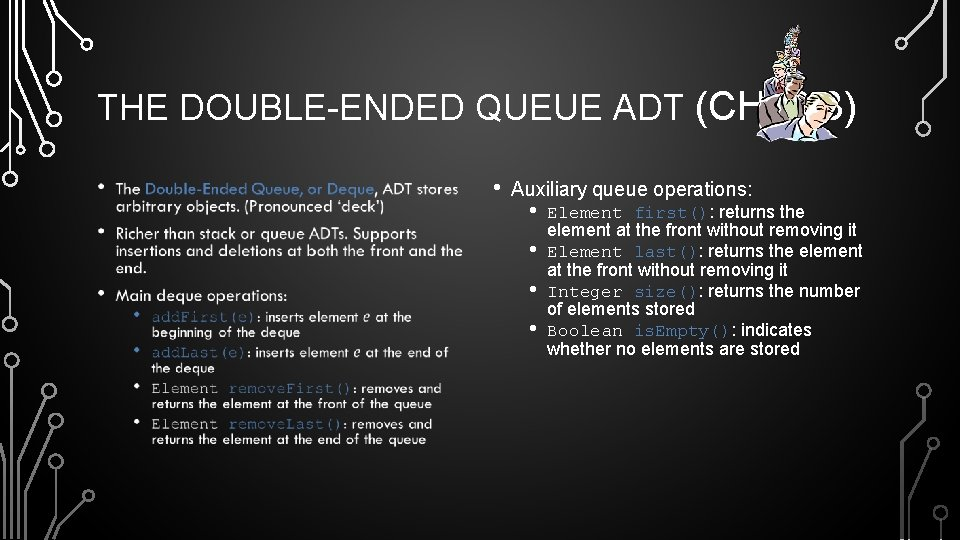THE DOUBLE-ENDED QUEUE ADT (CH. 6. 3) • • Auxiliary queue operations: • • Element first(): returns the element at the front without removing it Element last(): returns the element at the front without removing it Integer size(): returns the number of elements stored Boolean is. Empty(): indicates whether no elements are storedDEQUE WITH A DOUBLY LINKED LIST • last first elementsPERFORMANCE AND LIMITATIONS DOUBLY LINKED LIST IMPLEMENTATION •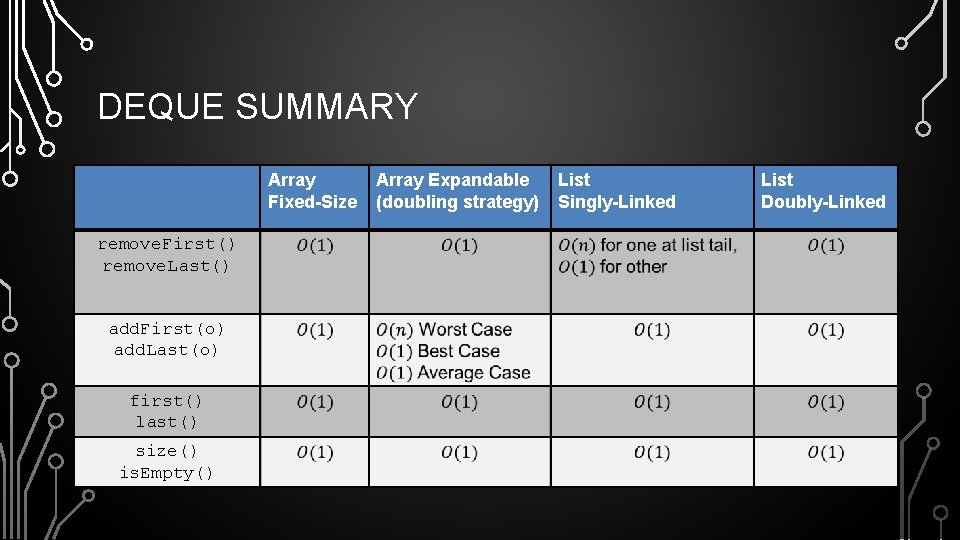DEQUE SUMMARY Array Fixed-Size remove. First() remove. Last() add. First(o) add. Last(o) first() last() size() is. Empty() Array Expandable (doubling strategy) List Singly-Linked List Doubly-Linked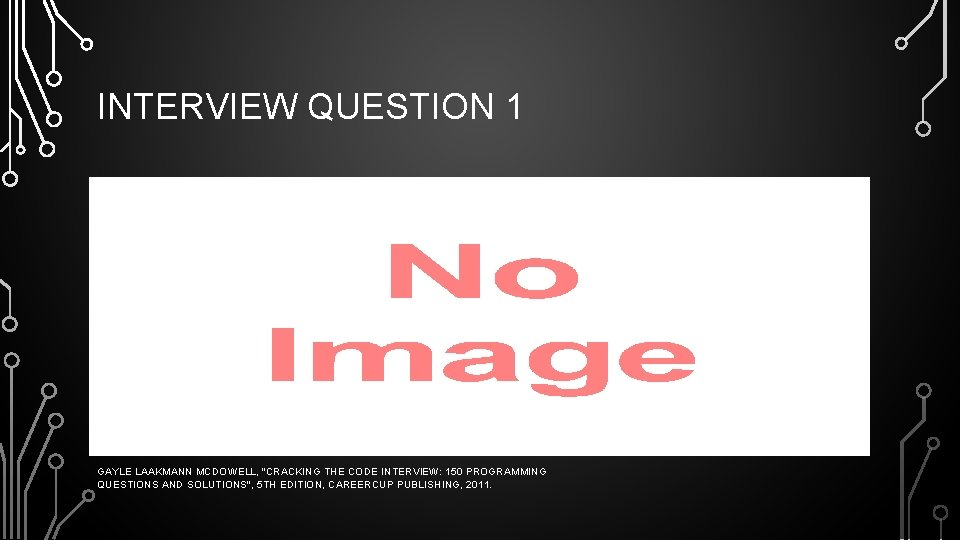INTERVIEW QUESTION 1 • GAYLE LAAKMANN MCDOWELL, "CRACKING THE CODE INTERVIEW: 150 PROGRAMMING QUESTIONS AND SOLUTIONS", 5 TH EDITION, CAREERCUP PUBLISHING, 2011.INTERVIEW QUESTION 2 • An animal shelter holds only dogs and cats, and operates in a strictly "first in, first out" basis. People must adopt either the "oldest" (based on arrival time) of all animals at the shelter, or they can select whether they prefer a dog or cat (and will receive the oldest animal of that type). They cannot select which specific animal they would like. Create the data structure(s) to maintain this system and implement operations such as enqueue, dequeue. Any, dequeue. Dog, and dequeue. Cat. GAYLE LAAKMANN MCDOWELL, "CRACKING THE CODE INTERVIEW: 150 PROGRAMMING QUESTIONS AND SOLUTIONS", 5 TH EDITION, CAREERCUP PUBLISHING, 2011.